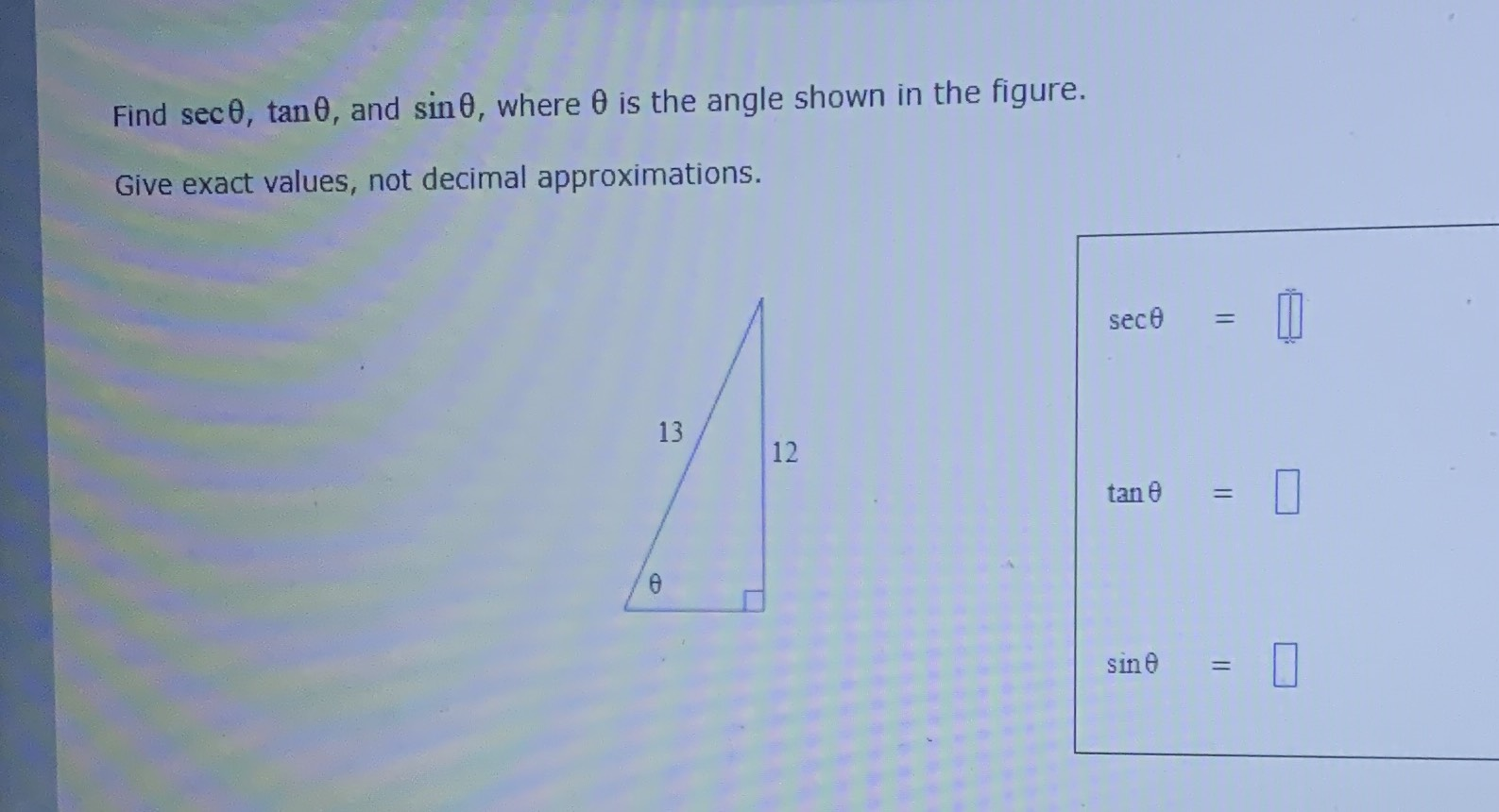### Still have math questions?Find $$\sec \theta , \tan \theta ,$$ and $$\sin \theta ,$$ where $$\theta$$ is the angle shown in the figure. Give exact values, not decimal approximations.
$$sec\theta= \frac{13}{5}\\\space \\tan\theta= \frac{12}{5}\\\space \\sin\theta= \frac{12}{13}$$